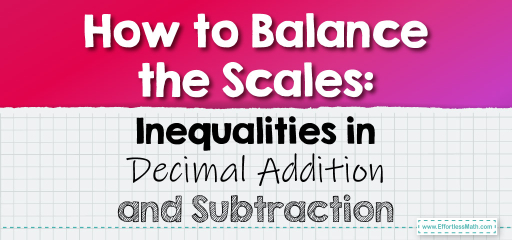# How to Balance the Scales: Inequalities in Decimal Addition and Subtraction

Inequalities are mathematical expressions that describe the relationship between two quantities. When working with decimals, inequalities can help us understand which of two quantities is larger or smaller, or if they are equal. Let's explore how to approach inequalities involving decimal addition and subtraction.Key Symbols:

– $$<$$: Less than

– $$>$$: Greater than

– $$=$$: Equal to

– $$\geq$$: Greater than or equal to

– $$\leq$$: Less than or equal to

## Inequalities in Decimal Addition and Subtraction

### Example 1: Evaluating an Addition Inequality

Is $$2.45 + 3.56 > 6$$?

Solution Process:

Add the decimals: $$2.45 + 3.56 = 6.01$$.

Since $$6.01 > 6$$, the inequality is true.

The Absolute Best Book for 5th Grade Students

### Example 2: Evaluating a Subtraction Inequality

Is $$7.89 – 4.44 < 3.5$$?

Solution Process:

Subtract the decimals: $$7.89 – 4.44 = 3.45$$.

Since $$3.45 < 3.5$$, the inequality is true.

Inequalities involving decimal addition and subtraction provide a deeper understanding of the relationships between quantities. By evaluating these inequalities, you can determine the relative sizes of different quantities, helping you make informed decisions and comparisons. Whether you’re comparing expenses, distances, or any other decimal values, understanding inequalities is a crucial skill. With practice, you’ll become adept at quickly evaluating and interpreting these relationships, making you a master of decimal dynamics!

### Practice Questions:

1. Determine if $$3.56 + 4.45 > 8$$.

2. Evaluate if $$9.01 – 5.67 < 3.5$$.

3. Is $$2.34 + 5.67 \leq 8.01$$?

4. Determine if $$8.90 – 4.45 \geq 4.5$$.

5. Evaluate if $$4.56 + 2.34 > 7$$.

A Perfect Book for Grade 5 Math Word Problems!

1. True ($$3.56 + 4.45 = 8.01$$)

2. True ($$9.01 – 5.67 = 3.34$$)

3. True ($$2.34 + 5.67 = 8.01$$)

4. True ($$8.90 – 4.45 = 4.45$$)

5. False ($$4.56 + 2.34 = 6.90$$)

The Best Math Books for Elementary Students

### What people say about "How to Balance the Scales: Inequalities in Decimal Addition and Subtraction - Effortless Math: We Help Students Learn to LOVE Mathematics"?

No one replied yet.

X
51% OFF

Limited time only!

Save Over 51%

SAVE $15 It was$29.99 now it is \$14.99